Courses

# Capacitance MSQ

## 10 Questions MCQ Test Topic wise Tests for IIT JAM Physics | Capacitance MSQ

Description
This mock test of Capacitance MSQ for Physics helps you for every Physics entrance exam. This contains 10 Multiple Choice Questions for Physics Capacitance MSQ (mcq) to study with solutions a complete question bank. The solved questions answers in this Capacitance MSQ quiz give you a good mix of easy questions and tough questions. Physics students definitely take this Capacitance MSQ exercise for a better result in the exam. You can find other Capacitance MSQ extra questions, long questions & short questions for Physics on EduRev as well by searching above.
*Multiple options can be correct
QUESTION: 1

### A parallel plate capacitor is charged from a cell and then isolated from it. The separation between the plates is now increased

Solution:

The correct answers are: The field in the region between the plates will not change, The energy stored in the capacitor will increase

*Multiple options can be correct
QUESTION: 2

### In the figure initial status of capacitance and their connection is shown. Which of the following is correct about this circuit :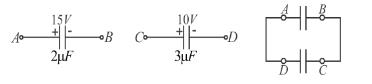Solution: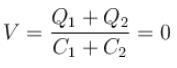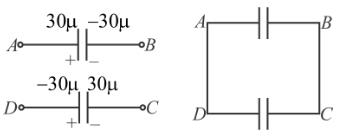Final potential difference = 0
Final charge = 0
Charge flow 30µc from A to D

The correct answers are: Final charge on each capacitor will be 0, Final total electrical energy of the capacitors will be 0, Total charge flown from A to D is 30µC

*Multiple options can be correct
QUESTION: 3

### Capacitor C1 of the capacitance 1μF and capacitor C2 of capacitance 2μF are separately charged fully by a common battery. The two capacitors are then separately allowed to discharge through equal resistors at time t = 0.

Solution:

The instantaneous charge on the capacitor is  q(t) = q0[1 – et/R C] = CV[1 – et/R C]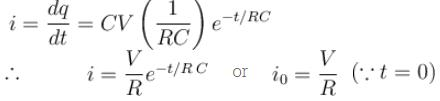The correct answers are: the current in the two discharging circuits at t = 0 are equal but non zero., capacitor C1 loses 50% of its initial charge sooner than C2 loses 50% of its initial charge

*Multiple options can be correct
QUESTION: 4

Two identical capacitors are charged to different potentials then they are connected to each other in such a way that the sum of charges of plates having positive polarity remains constant. Mark the incorrect statement.

Solution:

As the charge of isolated system remains conserved, so the sum of charges of plates having negative polarity remains constant. As potential of two capacitors are different so some charge flows into the circuit till both acquire the same potential.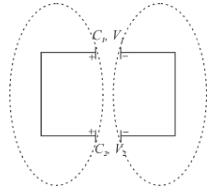As charge flows. ΔH ≠ 0,
Let final common potential be V, then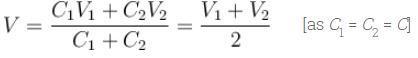The incorrect options are:

-Mean of individual final potentials is different from mean of individual initial potentials.

-Total energy stored in two capacitors in final state may be equal to that in initial state., -Heat disspation in the circuit could be zero.

*Multiple options can be correct
QUESTION: 5

A parallel plate capacitor of capacitance is connected to a cell of emf 10Volt and fully charged. Now a dielectric slab (k = 3) of thickness equal to the gap between the plates, is very slowly inserted to completely fill in the gap, keeping the cell connected. During the filling process:

Solution:

Charge on capacitor before insertion of dielectric slab = 100µC
Charge on capacitor after insertion of dielectric slab = 300µC
Increase in charge on the capacitor = 300 – 100 = 200µC
Heat produced = 0
Energy supplied by the cell = increase in stored potential energy + work done on the person who filling the dielectric slab + heat produced.

The correct answers are: the increase in charge on the capacitor is 200µC.   , the heat produced is 0., energy supplied by the cell = increase in stored potential energy + work done on the person who is filling the dielectric slab., energy supplied by the cell = increase in stored potential energy + work done on the person who is filling the dielectric slab + heat produced.

*Multiple options can be correct
QUESTION: 6

A parallel plate capacitor is charged and then the battery is disconnected. When the plates of the capacitor are brought closer, then

Solution:

The correct answers are: energy stored in the capacitor decreases, the potential difference between the plates decreases, the capacitance increases

*Multiple options can be correct
QUESTION: 7

The plates of a parallel plate capacitor with no dielectric are connected to a voltage source. Now a dielectric of dielectric constant K is inserted to fill the whole space between the plates with voltage source remaining connected to the capacitor.

Solution:

(A)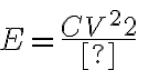As potential difference source between the plates is connected, potential difference remains constant. But capacitance C becomes KC hence energy stored is increased by factor K.
(B) Electric field  is not changed.
(C) Charge on each plate is increased by factor K hence force between them increases by factor K2.
For effect of the medium, they must completely lie in the medium.
(D) Q = CV
Hence charge becomes KQ as C becomes KC and V remain unchanged.

The correct answers are: the energy stored in the capacitor will become K-times, the force of attraction between the plates will become K2-times, the charge on the capacitor will become K-times.

*Multiple options can be correct
QUESTION: 8

A capacitor (without dielectric) is discharging through a resistor. At some instant a dielectric is inserted between the plates, then which of the following is not true.

Solution:

Let the capacitance before insertion of dielectric be C and the resistance be R.

∴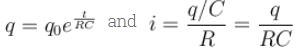∵ Just after insertion of dielectric the capacitance increases.
∴ The charge just after insertion of dielectric remains same, but the current decreases.

The energy stored in capacitor is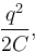hence energy decrease

The correct answers are: Just after the insertion of the dielectric, current will increase, Just after the insertion of the dielectric, charge on capacitor will increase., Just after the insertion of the dielectric, energy stored in the capacitor will increase.

*Multiple options can be correct
QUESTION: 9

A parallel plate capacitor of capacitance C has charges on its plates initially as shown in the figure. Now at t = 0, the switch S is closed. Select the correct alternative(s) for this circuit diagram.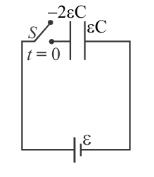Solution:

Suppose charge flown through the battery is Q, then charge distribution will be as :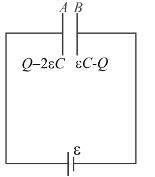The electric field in the region between and B is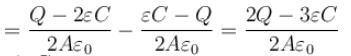∴  Potential difference between the plates,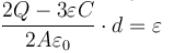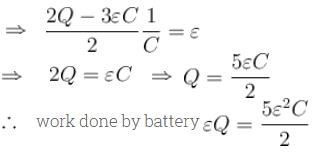The correct answers are: In steady state the charges on the outer surfaces of plates A and B will be same in magnitude and sign., In steady state the charges on the inner surfaces of the plates A and B will be same in magnitude and opposite in sign., The work done by the cell by the time steady state is reached is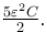*Multiple options can be correct
QUESTION: 10

The figure shows, a graph of the current in a discharging circuit of a capacitor through a resistor of resistance 10Ω.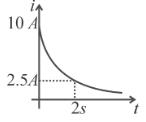Solution:

V0 = I0R = 10 × 10 = 100 volts (since, I0 = 10amp from figure)
Also : I = I0et/RC
Taking log :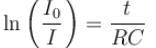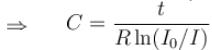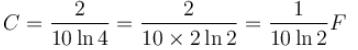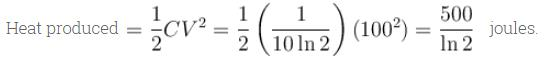Thermal power in the resistor will decrease with a time constant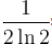second.
The correct answers are: The initial potential difference across the capacitor is 100volt., The capacitance of the capacitor is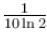Farad., The total heat produced in the circuit will be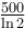Joules., The thermal power in the resistor will decrease with a time constant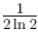second.